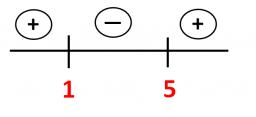# Difference 3539

We increased the unknown number by 29% once and 41% the second time. The difference between the new numbers was 420. What was the unknown number?

x =  3500

### Step-by-step explanation:

1.41·x-1.29·x = 420

0.12x = 420

x = 420/0.12 = 3500

x = 3500

Our simple equation calculator calculates it.Did you find an error or inaccuracy? Feel free to write us. Thank you!

Tips for related online calculators